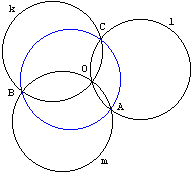# 3 circles having the same radius

Roger A. Johnson, a notable geometer and the author, among others, of a well known book Advanced Euclidean Geometry, posed in 1916 (Am Math Monthly, Vol. 23, No. 5. (May, 1916), pp. 161-162) a solution to an engaging problem:If three circles having the same radius pass through a point, the circle through their other three points of intersection also has the same radius. The circles are denoted k, l, m. The points of their intersection are 0, A, B, C.

Johnson believed that the problem was new, although under a little different guise it appeared a hundred years earlier in Mathematical Repository, volume IV (1819). Johnson's note was immediately followed by an article of Arnold Emch where the authored explored the link between that problem and the inversion in the incircle of a triangle.

Johnson's solution## SolutionDenote the centers of the circles Ck, Cl, Cm. Then OClACm is a rhombus, and so is OCmBCk. Hence, ClA and CkB are equal and parallel, CkClAB is a parallelogram, and AB is equal to CkCl. Thus the triangles CkClCm and ABC are congruent, and have equal circumcircles. But the circumcircle of the former has its center at O, and is equal to each of the given circles. Hence the circle through A, B, C is equal to each of the given circles.

Johnson also stated the following

### Corollary

Each of the four points O, A, B, C is the orthocenter of the triangle of the other three, and the set of points has all the well-known properties of an orthocentric system.As we already mentioned, Johnson's note was followed by a more expansive article by Arnold Emch who, besides observing the relevance to the problem of the inversion in the incircle of a triangle gave another elementary solution.

First of all, ∠OCB = ∠OAB, because the circles k and l are equal and have the common chord OB subtending those angles. Likewise, ∠OBC = ∠OAC. Consequently

 ∠OCB + ∠OBC = ∠OAB + ∠OAC = ∠BAC,

so that ∠BOC and ∠BAC are supplementary. But also ∠BKC and ∠BOC are supplementary, for any point K on k; hence ∠BAC = ∠BKC. From this follows immediately that the circle α through ABC is equal to the circle k, and consequently to l and m. As the sum of the six angles in the equality

 ∠OBA + ∠OBC + ∠OCB = ∠OCA + ∠OAC + ∠OAB

is equal to the sum of angles in the triangle ABC, the left and the right hand sides are equal to a right angle, i.e., BCQ is a right triangle (Q being the intersection of OC and AB). Consequently CQ is perpendicular to AB. Likewise BO and AO prolonged are perpendiculars to AC and CB, respectively; O is the orthocenter of triangle ABC. In a similar manner it can be shown, that A, B, C are orthocenters of the corresponding triangles OBC, OCA, OAB.### 3 Equal and Concurrent Circles

1. Johnson Circles
2. Three equal circles
3. 3 circles having the same radius
4. Inversionn in the Incircle
5. Orthocenter and Three Equal Circles